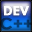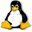Home Latest News Archive Tutorials C++ 2 3 4 Win32 Java OpenGL GLUT OpenGL ES 2 3 4 DirectX VB.NET HTML Photoshop 3dsmax Forums Projects Modelling Links About Us Search Recent Tutorials C / C++ Win32 OpenGL OpenGL ES GLUT## Dynamic Matrices

Tutorials > C++ > Dynamic Matrices

### Introduction

In the previous tutorial, we learnt about dynamic arrays. This tutorial will explain how to create dynamic matrices. A matrix is a 2-dimensional array. This was dealt with in tutorial 25. It was discussed then that a 2-dimensional array was essentially an array of arrays.

Contents of main.cpp :

```#include <iostream>
#include <stdlib.h>

using namespace std;

int main()
{
```

Firstly, the code below allows you to enter the number of rows and the number of columns you would like in the matrix.

```	int rowSize = 0;
int colSize = 0;

cout << "Please enter a row size : ";
cin >> rowSize;
cout << "Please enter a column size : ";
cin >> colSize;
```

Our next step is to create a pointer to a pointer to an integer. This may seem strange but you will see why this is necessary below.

```	int **matrix = NULL;
```

We first allocate an array of size rowSize. This array consists of pointers to integers shown by the new int*. Each pointer to an integer will point to their own arrays, each one representing a row. This is where the array of arrays is shown clearly.

```	matrix = new int*[rowSize];
```

The next step is to loop through all integer pointers and allocate each one an array of size colSize.

```	for (int i = 0; i < rowSize; i++)
matrix[i] = new int[colSize];
```

The matrix can then be used as per normal.

```	for (int i = 0; i < rowSize; i++)
for (int j = 0; j < colSize; j++)
matrix[i][j] = i * j % 10;

for (int i = 0; i < rowSize; i++)
{
for (int j = 0; j < colSize; j++)
cout << matrix[i][j] << " ";

cout << endl;
}
```

When reclaiming the memory, you must first deallocate all memory allocated to each row. Once that has been done you can free up memory from the original array of integer pointers.

```	for (int i = 0; i < rowSize; i++)
delete[] matrix[i];

delete[] matrix;

matrix = NULL;

system("pause");

return 0;
}```

Dynamic matrices appear in many applications ranging from grids and tables to images. You should now be able to create matrices with ease. In C, the idea is the same except that the malloc and free functions are used instead.

Please let me know of any comments you may have : Contact Me

 Source Files :< Tutorial 41 - Dynamic Arrays Tutorial 43 - String-Number Conversions >